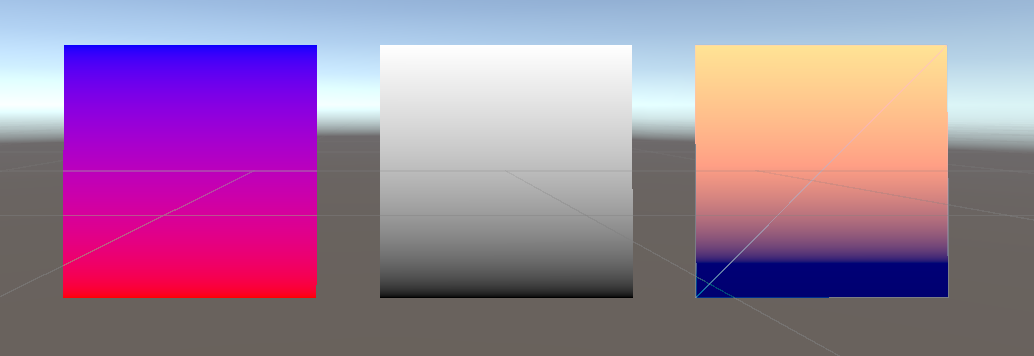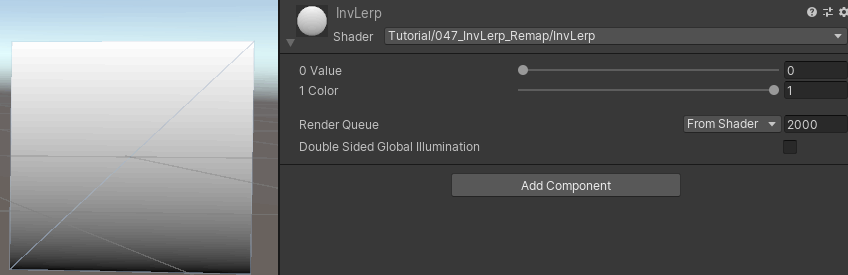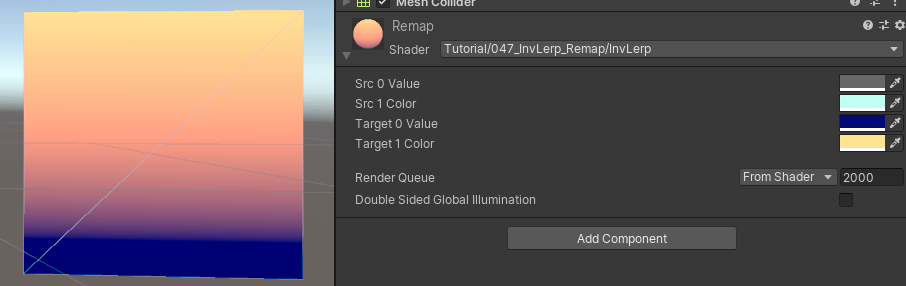In a previous tutorial I explained how the builtin `lerp` function works. Now I want to add the inverse lerp as well as the remap functions to this. They’re not builtin functions so we’ll have to write our own implementations. While this is a tutorial that focuses on explaining mathematical concepts, they resolve into basic addition and multiplication pretty quickly so I hope it isn’t too hard.The base shader is pretty barebones, a little more complex than a completely unlit one. I decided to write the custom functions in a separate include file which I named Interpolation.cginc, but you can just as well copy-paste the functions into your main shader file. As the “blending variable” I used the y component of the UV coordinates so it’s immediately visible what the function does over a gradient from 0 to 1.

A shader version for a regular linear interpolation looks like this:

``````Shader "Tutorial/047_InvLerp_Remap/Lerp"{
//show values to edit in inspector
Properties{
_FromColor ("From Color", Color) = (0, 0, 0, 1) //the base color
_ToColor ("To Color", Color) = (1,1,1,1) //the color to blend to
}

//the material is completely non-transparent and is rendered at the same time as the other opaque geometry
Tags{ "RenderType"="Opaque" "Queue"="Geometry"}

Pass{
CGPROGRAM

#include "UnityCG.cginc"
#include "Interpolation.cginc"

#pragma vertex vert
#pragma fragment frag

//the colors to blend between
fixed4 _FromColor;
fixed4 _ToColor;

//the object data that's put into the vertex shader
struct appdata{
float4 vertex : POSITION;
float2 uv : TEXCOORD0;
};

//the data that's used to generate fragments and can be read by the fragment shader
struct v2f{
float4 position : SV_POSITION;
float2 uv : TEXCOORD0;
};

v2f vert(appdata v){
v2f o;
//convert the vertex positions from object space to clip space so they can be rendered
o.position = UnityObjectToClipPos(v.vertex);
o.uv = v.uv;
return o;
}

fixed4 frag(v2f i) : SV_TARGET{
float blend = i.uv.y;
fixed4 col = lerp(_FromColor, _ToColor, blend);
return col;
}

ENDCG
}
}
}
``````

And the “barebones” include file looks like this, the `ifdef` and `define` statements are there to allow including the file multiple times over multiple files without leading to errors.

``````//avoid multiple imports
#ifndef INTERPOLATION
#define INTERPOLATION

/* //hlsl supports linear interpolation intrinsically so this isn't needed
float lerp(float from, float to, float rel){
return ((1 - rel) * from) + (rel * to);
}
*/

#endif
``````

## Inverse Lerp

Lerp does a interpolation that returns values between the `from` and `to` input values for interpolation values between 0 and 1. The inverse of that is a function which we can hand a third value and it’ll return how close that value is to the first or second value.

``````float inverseLerped = invLerp(from, to, value);
float result = lerp(from, to, inverseLerped);
``````

In this example the `result` should always be the same value as the input `value`. Similarly first doing a lerp and then an inverse lerp with the arguments chained like this shouldn’t change anything.

I like the straightforward way we can deduce the function. We start by making sure that if the value is the same as the lower bound, the function returns a `0`, we do this by subtracting the `from` variable from the value.

``````float invLerp(float from, float to, float value){
return value - from;
}
``````

With this setup the function returns 0 when the input value is equal to the from variable. Next let’s ensure that a input value equal to the to variable results in a output of `1`. So far the output would be `from - to`, so lets divide the whole thing we wrote so far by `from - to`. With this the function is already done.

``````float invLerp(float from, float to, float value){
return (value - from) / (to - from);
}
``````

With this you can can get gradients in a 0 to 1 range from any other gradient. You could replace all of the arguments with multidimensional vectors (`float2`, `float3`, `float4`), but it’s far less useful than with `lerp` unless you want a component-wise inverse lerp.The `smoothstep` function that’s built into hlsl does almost the same as our inverse lerp function, but it also applies cubic smoothing, so it’s marginally more expensive to calculate and only works between 0 and 1 while our function can also extrapolate. It’s best to try around with both to get a feel for which to use for which occasion. (I admit I use smoothstep a lot when invLerp would be better, just because I don’t have to add the function to the project…)

## Remap

I mentioned earlier that chaining inverse lerp and lerp with the same arguments results in no change. While this is still true, we can chain them with different arguments for the lower and upper bounds. The custom remap function I wrote takes 5 arguments, the source bounds as well as the target bounds and the original value. The “remap” action then remaps those values so the a original value of the source `from` value will become a target `from` value. Similarly the `to` values and those inbetween. This allows you to remap linear gradients however you want.

``````float remap(float origFrom, float origTo, float targetFrom, float targetTo, float value){
float rel = invLerp(origFrom, origTo, value);
return lerp(targetFrom, targetTo, rel);
}
``````

This also has more of a use with vectors because it can be used to set the whitelevel of a color output. After also creating the same vector version for the invLerp function you can create a multidimensional version by replacing all `float` with the fitting vector version.## Sources

``````//avoid multiple imports
#ifndef INTERPOLATION
#define INTERPOLATION

/* //hlsl supports linear interpolation intrinsically so this isn't needed
float lerp(float from, float to, float rel){
return ((1 - rel) * from) + (rel * to);
}
*/

float invLerp(float from, float to, float value) {
return (value - from) / (to - from);
}

float4 invLerp(float4 from, float4 to, float4 value) {
return (value - from) / (to - from);
}

float remap(float origFrom, float origTo, float targetFrom, float targetTo, float value){
float rel = invLerp(origFrom, origTo, value);
return lerp(targetFrom, targetTo, rel);
}

float4 remap(float4 origFrom, float4 origTo, float4 targetFrom, float4 targetTo, float4 value){
float4 rel = invLerp(origFrom, origTo, value);
return lerp(targetFrom, targetTo, rel);
}

#endif
``````
``````Shader "Tutorial/047_InvLerp_Remap/InvLerp"{
//show values to edit in inspector
Properties{
_SrcZeroValue ("Src 0 Value", Color) = (0,0,0,1) //source min value
_SrcOneValue ("Src 1 Color", Color) = (1,1,1,1) //source max value
_TargetZeroValue ("Target 0 Value", Color) = (0,0,0,1) //target min value
_TargetOneValue ("Target 1 Color", Color) = (1,1,1,1) //target max value
}

//the material is completely non-transparent and is rendered at the same time as the other opaque geometry
Tags{ "RenderType"="Opaque" "Queue"="Geometry"}

Pass{
CGPROGRAM

#include "UnityCG.cginc"
#include "Interpolation.cginc"

#pragma vertex vert
#pragma fragment frag

//the colors to blend between
fixed4 _SrcZeroValue;
fixed4 _SrcOneValue;
fixed4 _TargetZeroValue;
fixed4 _TargetOneValue;

//the object data that's put into the vertex shader
struct appdata{
float4 vertex : POSITION;
float2 uv : TEXCOORD0;
};

//the data that's used to generate fragments and can be read by the fragment shader
struct v2f{
float4 position : SV_POSITION;
float2 uv : TEXCOORD0;
};

v2f vert(appdata v){
v2f o;
//convert the vertex positions from object space to clip space so they can be rendered
o.position = UnityObjectToClipPos(v.vertex);
o.uv = v.uv;
return o;
}

fixed4 frag(v2f i) : SV_TARGET{
float blend = i.uv.y;
fixed4 col = remap(_SrcZeroValue, _SrcOneValue, _TargetZeroValue, _TargetOneValue, blend);
return col;
}

ENDCG
}
}
}
``````
``````Shader "Tutorial/047_InvLerp_Remap/Remap"{
//show values to edit in inspector
Properties{
_SrcZeroValue ("Src 0 Value", Color) = (0,0,0,1) //source min value
_SrcOneValue ("Src 1 Color", Color) = (1,1,1,1) //source max value
_TargetZeroValue ("Target 0 Value", Color) = (0,0,0,1) //target min value
_TargetOneValue ("Target 1 Color", Color) = (1,1,1,1) //target max value
}

//the material is completely non-transparent and is rendered at the same time as the other opaque geometry
Tags{ "RenderType"="Opaque" "Queue"="Geometry"}

Pass{
CGPROGRAM

#include "UnityCG.cginc"
#include "Interpolation.cginc"

#pragma vertex vert
#pragma fragment frag

//the colors to blend between
fixed4 _SrcZeroValue;
fixed4 _SrcOneValue;
fixed4 _TargetZeroValue;
fixed4 _TargetOneValue;

//the object data that's put into the vertex shader
struct appdata{
float4 vertex : POSITION;
float2 uv : TEXCOORD0;
};

//the data that's used to generate fragments and can be read by the fragment shader
struct v2f{
float4 position : SV_POSITION;
float2 uv : TEXCOORD0;
};

v2f vert(appdata v){
v2f o;
//convert the vertex positions from object space to clip space so they can be rendered
o.position = UnityObjectToClipPos(v.vertex);
o.uv = v.uv;
return o;
}